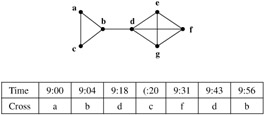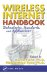# 11.2 Preliminaries

## 11.2 Preliminaries

### 11.2.1 Movement History

We will assume that the user's location is given in symbolic coordinates, and that the system has a record of the user's past movements based on its location updates. The user's movement history is thus represented as a sequence of symbols from an alphabet A, which is finite. The information contained in the record depends on the system's update scheme. How this information is interpreted affects also the results of prediction algorithms.

For example, in movement-based update schemes, updates occur every time the user has crossed M cell boundaries. ,  If M = 1, then a record can look like the table in Figure 11.1, which has the details of all the user's cell crossings of the map on the left from 9 a.m. to 10 a.m.Figure 11.1: Example of a Cell Boundary Graph and Movement History

In this case, each symbol of the sequence is an ordered pair (t, v), where t is the time of update (and is discretized) and v is the user's new location. Depending on the purpose of a prediction algorithm, this sequence may be transformed to a new one so that the size of A is smaller.  For instance, if a prediction algorithm's objective is to predict the user's next cell, it may just consider the sequence abdefdb. In this case, A = V, the set of all the cell IDs.

For a time-based update scheme such as described in Rose,  updates occur every T time units. If we set T = 5 minutes, then the sequence generated from Figure 11.1 would be abbbeeeffdddb. Notice that while this sequence is able to capture the duration of residence of the user at a cell, it misses the cell crossings that took place between updates. It will, nonetheless, be useful for a prediction algorithm that seeks to predict the user's location in the next T time units.

In Bhattacharya and Das,  the authors suggested generating a movement history that reflects both cell crossings and durations of residence of the user at each cell, while keeping A = V. Such a history is generated when a user updates every T time units and every M cell crossings. If T = 5 and M = 1, the sequence that reflects the movement in Figure 11.1 would be abbbbdeeefffddddb.

Hence, there are different ways of representing a user's movement history as a sequence from a finite alphabet. It is imperative that the choice of sequence be matched to the purpose(s) of the prediction algorithm.

In the following discussion, we will assume that the appropriate movement history has been chosen. A history of length n is denoted as a sequence

Hn=X1 = a1, ..., Xn = an

where each Xi is a random variable and each ai A. For brevity we will sometimes denote a sequence as a1a2 ... an. The notation P(Xi = ai) denotes the probability that Xi takes the value ai anddenotes an estimate of P(Xi).

### 11.2.2 Approach

We will discuss various prediction algorithms which use different approaches to predict the next term of the user's itinerary, i.e., sequence L. When possible, we discuss also how these methods can be extended to predict not just the next term but the future terms of the sequence as well.

Prediction algorithms usually consist of two steps: (1) to assign conditional probabilities to the elements of A given the user's movement history H, and (2) to use these values to predict the next term in the sequence.

We note that there are some applications where a single guess for the next term may be too restrictive. For example, to satisfy QoS requirements, Chan and coworkers  proposed an algorithm that outputs a subset of A. so that the probability that the next term of the movement history is in this set is above some threshold.

Akyildiz, I., Ho, J., and Lin, Y., Movement based location update and selective paging for PCS networks, IEEE/ACM Trans. Networking, 4 (4), 629–638, 1996.

Bar-Noy, A., Kessler, I., and Sidi, M., Mobile users: to update or not to update?, ACM/Baltzer J. Wireless Networks, 1 (2), 175–195, 1995.

Intuitively, the smaller |A| is, the better because there will be fewer choices for a prediction.

Rose, C., Minimizing the average cost of paging and registration: a timer-based method, Wireless Networks, 2 (2), 109–116, 1996.

Bhattacharya, A. and Das, S.K., LeZi-update: an information-theoretic framework for personal mobility tracking in PCS networks, ACM/Kluwer Wireless Networks, 8 (2–3), 121–135, 2002.

Chan, J., Zhou, S., and Seneviratne, A., A QoS adaptive mobility prediction scheme for wireless networks, Proc. IEEE Globecom, Sydney, Australia, Nov. 1998.Wireless Internet Handbook: Technologies, Standards, and Applications (Internet and Communications)
ISBN: 0849315026
EAN: 2147483647
Year: 2003
Pages: 239